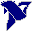# LabVIEW

cancel
Showing results for
Did you mean:

## Problem with Derivative x(t).VI

The "Derivative x(t).VI" works when I select any method but "4th Order Central" using the default Inital and Final Conditions. The 4th Order produces an:

error -2006   "Analysis:  The number of samples must be >= 2."

The number of samples is 1000. The VI also produces the same error if I provide a data array to the Initial and Final Condition rather than using the default values. Am I doing something wrong? Is it a bug?

Message 1 of 5
(1,431 Views)

## Re: Problem with Derivative x(t).VI

I'm using LabVIEW 2014 Version 14.0.f1 (32-bit)

Message 2 of 5
(1,423 Views)

## Re: Problem with Derivative x(t).VI

Hi jarniva,

please read the help for the derivative function. It mentions some boundary conditions for the "4th order" calculation you need to supply!

Best regards,
GerdWusing LV2011SP1 + LV2017 (+LV2020 sometimes) on Win10+cRIO
Message 3 of 5
(1,421 Views)

## Re: Problem with Derivative x(t).VI

I did read the help file. It says that the initial and final conditions minimize the error at the boundaries. I assumed that the default conditions woud work the same for both the 2nd order and 4th order methods. 2nd order works perfectly. I tried wiring a constant array for the boundary conditions and got the same error. What is different between the two methods?

Message 4 of 5
(1,416 Views)

## Re: Problem with Derivative x(t).VI

Hi jamiva,

to quote the LabVIEW help for Derivative:

Initial Condition: If method is 4th Order Central, the VI uses the first two elements in Initial Condition to calculate the derivative. The default is .
Final Condition: If method is 4th Order Central, the VI uses the first two elements in Final Condition to calculate the derivative. The default is .

Again: Did you read the help for that function? Did you read all of the help? Do you understand what it says?

Best regards,
GerdWusing LV2011SP1 + LV2017 (+LV2020 sometimes) on Win10+cRIO
Message 5 of 5
(1,375 Views)